# Teaching Equivalent Fractions

 Listen to this Lesson:

When learning about equivalent fractions, students learn how to represent equivalent fractions using area models, as well as visualize equivalent fractions using number lines. They also become fluent in determining equivalent fractions.

Whether you’re a math teacher or a homeschooling parent, you can turn your lesson on equivalent fractions into a success by blending theory with fun activities! To this end, in this article, we bring you a few teaching ideas, as well as practical activities. Read on to learn more.## Strategies to Teach Equivalent Fractions

### What Are Fractions?

Start your lesson on ration equivalence by defining fractions. We use fractions to represent a part of a whole. They are made up of a numerator on top and a denominator on the bottom. The numerator and denominator are separated by a horizontal line called a ‘fraction bar’.

Present a drawing of a fraction on the whiteboard, and illustrate the numerator and denominator in it. For instance, you can present 3⁄4 by shading three parts out of four in a circle. Point out that 3 is the numerator in this fraction, whereas 4 is the denominator.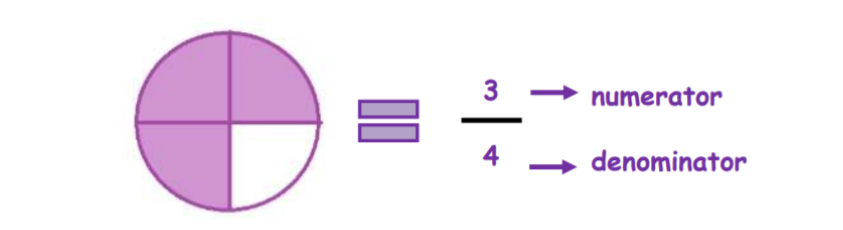Explain that the numerator represents ‘the part of a whole’ and the denominator represents ‘the total of the whole’. That’s why in the above drawing, as there are three shaded parts and four total parts; the fraction representing the shaded parts is equal to 3⁄4 or ‘three-fourths’.

Provide another example. For instance, draw a figure where the shaded parts can be represented as 4⁄6 or ‘four-sixths’ and the un-shaded parts can be represented as or ‘two-sixths’. Point out that in this case, 4 is the numerator and 6 is the denominator.### What Are Equivalent Fractions?

Write the word ‘equivalence’ on the whiteboard. There’s a chance that some students have already come across this word. Ask them if they know the meaning of it. Does this word sound similar to any other word they know?

Some students might observe that ‘equivalence’ reminds them of the word ‘equal’ or ‘equality’. Then, explain that that equivalence refers to the state or fact of being equal in amount, quantity, value, etc.

Point out that the same applies to fractions – equivalent fractions are simply equal fractions, even though they look different.

More specifically, equivalent fractions are fractions that are equal and represent the same parts but have different values for their numerators and denominators. Provide an example, by drawing three figures representing three equivalent fractions, such as: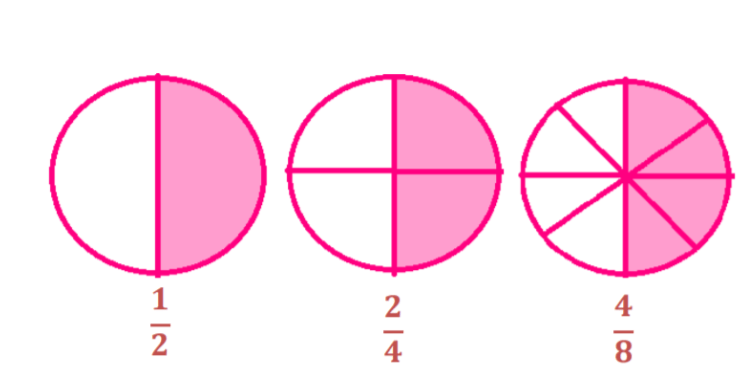Ask students to observe the three circles. Each circle is shaded exactly the same amount. But when written as fractions, these are expressed as 1⁄2, 2⁄4 and 4⁄8. These fractions represent the same amount of parts, so they are equivalent fractions.

### Representing Equivalent Fractions in a Number Line

Explain that we can express and represent fractions on a number line. We can also use the number line to find the equivalent fraction of 4⁄5 that has a denominator of 10. In other words, a number line can help if we’re trying to solve the following: 4⁄5= ?⁄10

Draw a number line on the whiteboard to illustrate this. Explain that first, we’ll divide the number line into 10 segments since we have a denominator of 5 and 10:Point out that we’ll arrange 1⁄5, 2⁄5, 3⁄5, 4⁄5 and 5⁄5 on the number line. Then, do the same for fractions with a denominator of 10:Ask students to look for the fraction with 10 as the denominator that aligns with 4⁄5. Point out that we can observe that 4⁄5= 8⁄10.

### How to Find Equivalent Fractions Using Multiplication

Once students understand the concept of equivalent fractions, they will find it easy to grasp how to find equivalent fractions, as well. Point out that we can do this by multiplication or division, which we’ll look into separately.

Explain to students that we can find equivalent fractions of a given fraction by multiplying the same number to its numerator and denominator. This is because when we multiply the numerator and denominator by the same number, the value of the fraction doesn’t change.

#### Example:

For instance, let’s say we want to find the equivalent fractions of 1⁄2. Point out that we’ll select a few random numbers and multiply the top and bottom part of this fraction by them. Let’s say that these numbers are 2, 3, 4, and 5. This will result in the following:

###How to Find Equivalent Fractions Using Division

Explain to students that we can also find equivalent fractions of a given fraction by dividing both the numerator and the denominator by the same number. We usually do this when we want to reduce the given fraction to a smaller equivalent fraction.

#### Example:

For instance, let’s say we want to find the equivalent fractions of 24/48. Point out that we’ll select a few random numbers by which the denominator and numerator are divisible and divide them by these numbers. Let’s say that these numbers are 2, 3, 4, and 6. This will result in the following:

###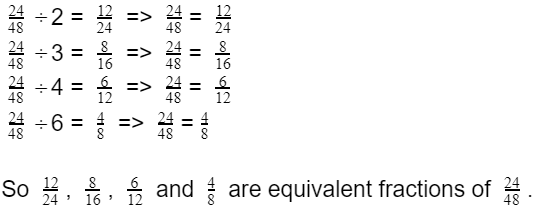Finding Missing Values in Equivalent Fractions Using Common Factors

Explain to students that when we need to find the missing numerator of an equivalent fraction, we can do this by finding the common factor shared by the denominators. Remind students that factors are numbers that we multiply to obtain another number (ex: 3 and 4 as factors of 12).

For instance, let’s say that we want to find the missing numerator in the following fraction: 3⁄7= ?⁄14. Point out that first, we’ll determine the factor we need in order to change 7 to 14. As 14 divided by 7 is 2, this means we’ll need to multiply 7 by 2 so that we end up with 14.

In order for the value of the fraction to remain unchanged, we’ll have to multiply the numerator, that is, 3, by the same number by which we multiply the denominator, i.e. 2. This will produce the following: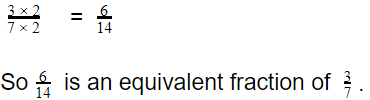Add that sometimes, there are instances when we’ll be dividing the two fractions by a common denominator as well. This usually occurs when we want to reduce the given fraction to its simplest form. Provide an example of such a case:Point out that we can observe that to get 12 from 48, we need to divide 48 by 4, because 48 divided by 12 is 4. We also know that in order for the fraction value to remain unchanged, we need to divide the numerator by the same number as the numerator, i.e. 4: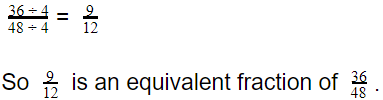If you have the technical possibilities in your classroom, you can also use multimedia materials, such as videos to enrich your lesson on equivalent fractions. Videos can be especially beneficial for the visualization of shaded figures with equivalent fractions.

For example, use this video to introduce the concept of equivalent fractions and illustrate to students how same-sized bars represent equal fractions with different values. This video is also a great resource, containing a fun pizza illustration on equivalent fractions.

This video contains simple guidelines on how to find equivalent fractions using multiplication, as well as worked examples. Finally, use this video on finding equivalent fractions through division, that is, by finding the common factor shared by the denominators.## Activities to Practice Equivalent Fractions

### Missing Numerator Game Board

This is a fun and interactive game that allows students to practice their skills at finding equivalent fractions using multiplication or division when there is a missing numerator in a given fraction.

Although the game requires a bit of prep work, it will definitely be worth your while as students are guaranteed to enjoy it. To use this activity in your class, you’ll need paper, markers, game figurines, and scissors.

Draw a game board resembling a winding road divided into equal spaces in the shape of mini-squares. Color each square with a different color. In each purple square, write ‘go back 5’. Include a ‘start’ and ‘finish’ square. Create as many game boards as needed for your class.#### Launch the Game!

Divide students into groups of 4 and hand out one game board per group, one answer sheet, 3 game figurines, and 20 task cards. In each group, there are 3 players and one checker that holds the answer key.

Provide instructions for the game. Point out that the 3 players compete against each other to reach the end of the winding road. They draw a card from the pile of cards and try to find the missing numerator using multiplication or division.

If they answer correctly, they can move their figurine one square on the game board. If they answer incorrectly, their figurines stay on the same square. All figurines are on the ‘start’ square when students start playing.

Each time a student answers, the designated checker in each group checks the answer sheet. The winner is the person that reaches the ‘finish’ square first. In the next round, the winner is the checker, whereas the current checker joins as a player.

### Equivalent Fractions Bingo

Equivalent Fractions Bingo is a great online game that will help students practice their understanding and recognition of fractions that are equivalent. To implement the game in your classroom, make sure there are enough technical devices for all students.

Students play the game individually, which makes this a suitable game for parents who are homeschooling their children, as well. Students are presented with a chart with several fractions and asked to find the equivalent fraction of a given fraction from the chart.

If students manage to answer the questions correctly and find the equivalent fractions, they get bingo! Play as many rounds as time allows.

### Pizza Fractions Game

Pizza Fractions Game is an awesome game that will have students coloring and practicing their knowledge of equivalent fractions. To use this game in your class, you’ll need some colorful paper, drawing pencils, and scissors.

Draw two pizzas representing two equivalent fractions, such as 1⁄2 and 2⁄4. The first pizza has two slices, whereas the second pizza has four slices. Use the drawing pencils to shade one slice or half of the pizza that represents 1⁄2 and leave the other pizza unshaded.

Cut out the two matching pizzas and create as many such matching pizzas as needed, depending on the size of your class. You can also choose to laminate the pizzas and re-use them next year.

Divide students into groups of 3, 4 and hand out the pizza fractions. Provide instructions for the game and tell students that they need to match as many pizzas as possible. Once they find a match, they also color the matching fraction in the pizza.

Provide a few minutes for students to finish this. Once each group is done, allow room for discussion and reflection. How did students determine how many parts to shade? Did they use multiplication or division for this purpose?

### Find Equivalent Fractions Game

This stimulating online game blends magic with learning and offers students the possibility to practice finding equivalent fractions. The only materials needed to implement this game in your classroom are a number of technical devices to play the game on.

This is an individual game, so homeschooling parents can also benefit from it. In this game, students have to put in jellies in the right quantity. By doing so, they are gathering the magic potion that’s in the jellies in the water and practicing equivalent fractions.

Provide a few minutes for students to complete the game. Then allow time for discussion and reflection in class. How can visual models help us determine which fractions are equal in value but look different?

## Before You Leave…

If you enjoyed these ideas and activities for teaching equivalent fractions, you’ll want to check out our lesson that’s dedicated to this topic! So if you need guidance to structure your class and teach it, sign up for our emails to receive loads of free content!

Feel free to also check out our blog – you’ll find plenty of awesome resources that you can use in your class! And if you’re ready to become a member, simply join our Math Teacher Coach community!